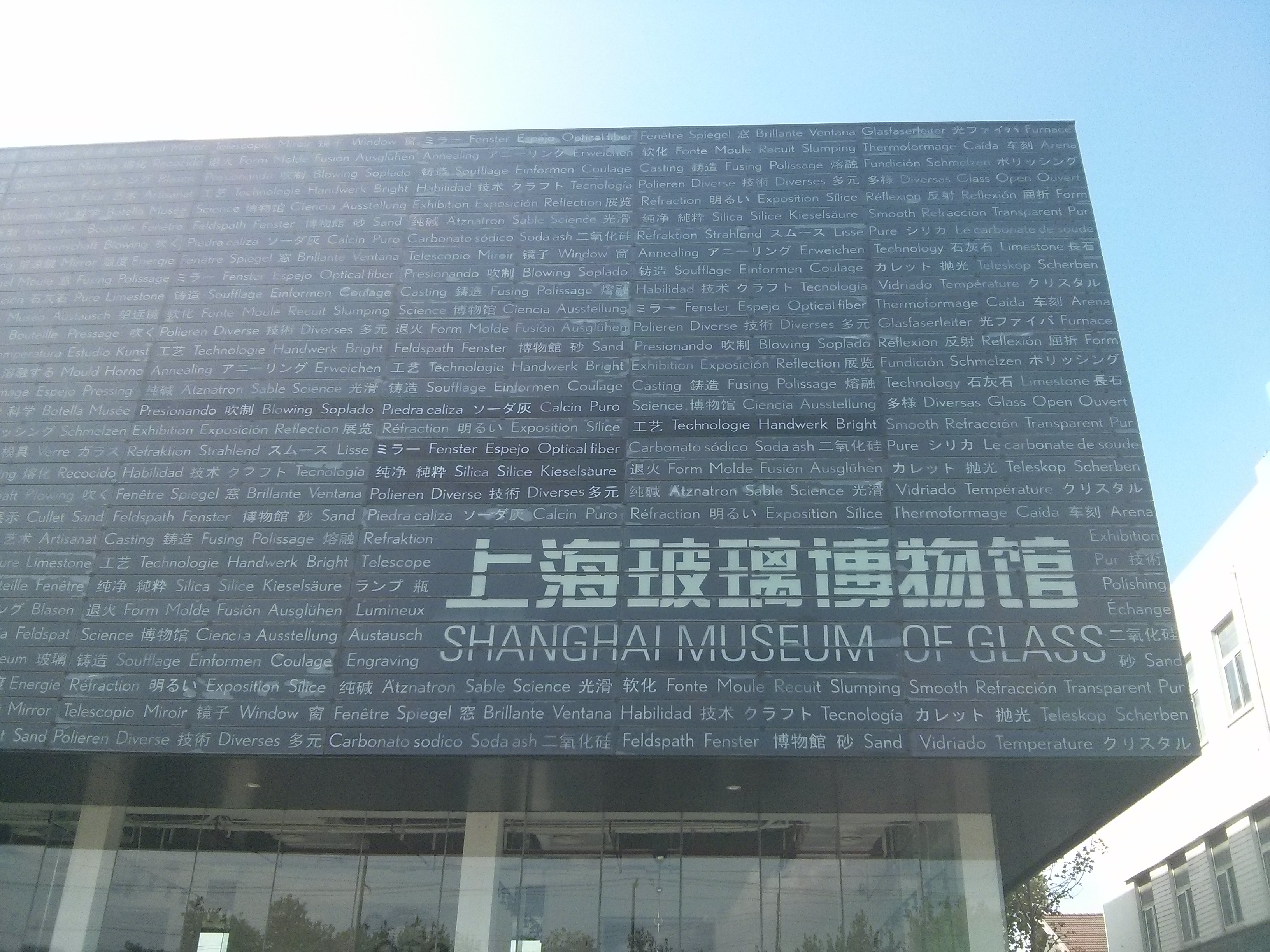# What if beyond what-if —— Spiders vs. the Sun

$\int_0^{2\pi}d\phi \int_0^{\frac{\pi}{2}}d\theta \sin\theta\int_0^{c\sqrt{\cos\theta}}r^2 dr=\frac{4\pi c^{3}}{15}$

$g_{\text{spider}}=\int_0^{2\pi}d\phi \int_0^{\frac{\pi}{2}}d\theta \sin\theta\int_0^{C_0}\cos\theta c^2\sqrt{\cos\theta}\frac{\rho G}{c^2}dc=\frac{4\pi\rho GC_0}{5}$

$=1.04\times 10^5\text{m/s}^2$

$6.67384\times 10^{-11}\times 1.989\times 10^{30}/(1.49\times10^{11})^2=0.0059\text{m/s}^2$

# 读后感——《管惟炎口述歷史回憶錄》

1. 共产党对于文人的控制和迫害远远超过了科学家和工程师，除了思想控制的需要外，毛泽东本身也是一个重理轻文的人，隔三差五就要找个文人吊上路灯批判一番。而物理所这样的地方则是受到了周恩来不小的保护，文革中受到的冲击也比较小。

2. 因为留学苏联，他错过了反右运动。而林昭就是在这项运动中醒了过来，进而一步步收到迫害。管惟炎觉悟的很晚，或者说他和共产党的割裂也从没有到达过林昭那种程度（大概也是因为他没怎么吃到过苦头吧），在文革中他也就可以说比较少的违心话就能过关。能够在对知识分子摧残最大的两次政治风波中置身事外，幸运女神真的很眷顾他。

3. 在零下271摄氏度的低温下，氦是没有摩擦力的，迷人的物理也让他可以暂时的忘掉人与人之间的摩擦，投入到对低温物理的钻研上去。对他来说，物理学就是一个避风港。去国之后，很多民运人士在异国他乡身无长技，而且在马列主义斗争哲学中浸淫多年，到了自由的土地上不由得“赢得了天空，失去了大地”，过的十分失意。而精于学术的管惟炎先生和⬤励之先生一样，在异国他乡依然可以成为受人尊敬的大学教授，过着体面而充实的生活。在国立清华大学，他还获得过每年由学生推选的杰出教学奖，更是十分不易。

# 弃子

“我杀了我们的孩子，现在它回来了，要杀掉我们所有人。”

# 第二天下午# 第四天……